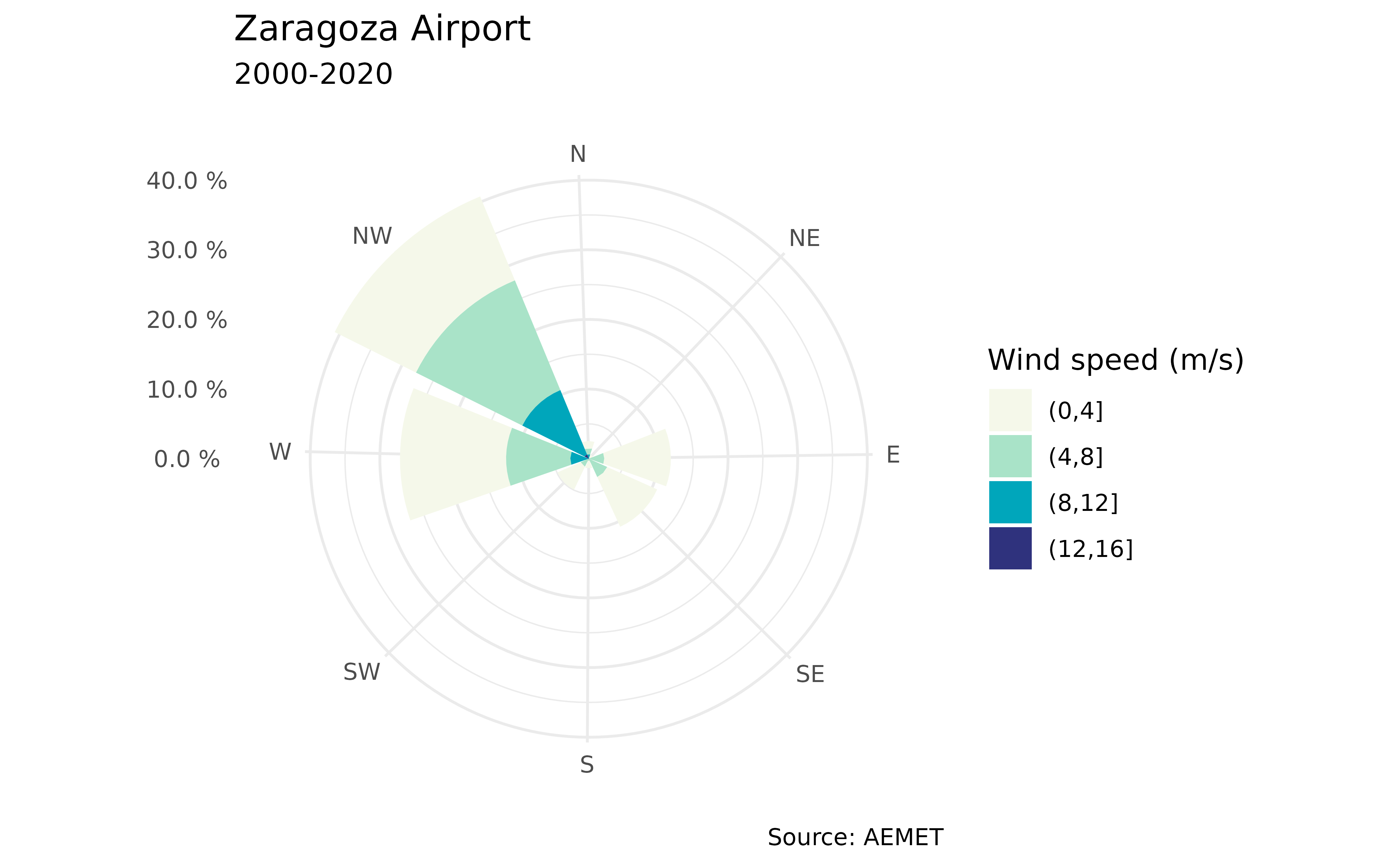Plot a windrose showing the wind speed and direction using ggplot2.

## Usage

ggwindrose(
speed,
direction,
n_directions = 8,
n_speeds = 5,
speed_cuts = NA,
col_pal = "GnBu",
legend_title = "Wind speed (m/s)",
calm_wind = 0,
n_col = 1,
facet = NULL,
plot_title = "",
...
)

## Arguments

speed

Numeric vector of wind speeds.

direction

Numeric vector of wind directions.

n_directions

Numeric value as the number of direction bins to plot (petals on the rose). The number of directions defaults to 8.

n_speeds

Numeric value as the number of equally spaced wind speed bins to plot. This is used if speed_cuts is NA (default 5).

speed_cuts

Numeric vector containing the cut points for the wind speed intervals, or NA (default).

col_pal

Character string indicating the name of the hcl.pals() color palette to be used for plotting.

legend_title

Character string to be used for the legend title.

calm_wind

Numeric value as the upper limit for wind speed that is considered calm (default 0).

n_col

The number of columns of plots (default 1).

facet

Character or factor vector of the facets used to plot the various windroses.

plot_title

Character string to be used for the plot title.

...

further arguments (ignored).

A ggplot2 object

## API Key

You need to set your API Key globally using aemet_api_key().

ggplot2::theme() for more possible arguments to pass to ggwindrose.

Other aemet_plots: climatestripes_station(), climatogram_normal(), climatogram_period(), ggclimat_walter_lieth(), ggstripes(), windrose_days(), windrose_period()

Other wind: climaemet_9434_wind, windrose_days(), windrose_period()

## Examples



library(ggplot2)

speed <- climaemet::climaemet_9434_wind$velmedia direction <- climaemet::climaemet_9434_wind$dir

rose <- ggwindrose(
speed = speed,
direction = direction,
speed_cuts = seq(0, 16, 4),
legend_title = "Wind speed (m/s)",
calm_wind = 0,
n_col = 1,
plot_title = "Zaragoza Airport"
)
rose + labs(
subtitle = "2000-2020",
caption = "Source: AEMET"
)